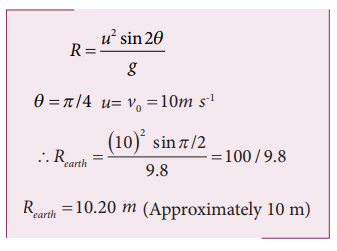Home | | Physics 11th std | Solved Example Problems for Projectile Motion

# Solved Example Problems for Projectile Motion

Physics : Kinematics : Projectile Motion

## Solved Example Problems for Projectile Motion

Example 2.37

Suppose an object is thrown with initial speed 10 m s-1 at an angle π/4 with the horizontal, what is the range covered? Suppose the same object is thrown similarly in the Moon, will there be any change in the range? If yes, what is the change? (The acceleration due to gravity in the Moon gmoon = 1/6 g)

### Solution

In projectile motion, the range of particle is given by,If the same object is thrown in the Moon, the range will increase because in the Moon, the acceleration due to gravity is smaller than g on Earth,The range attained on the Moon is approximately six times that on Earth.

### Example 2.38

In the cricket game, a batsman strikes the ball such that it moves with the speed 30 m s-1  at an angle 30o with the horizontal as shown in the figure. The boundary line of the cricket ground is located at a distance of 75 m from the batsman? Will the ball go for a six? (Neglect the air resistance and take acceleration due to gravity g = 10 m s-2).### Solution

The motion of the cricket ball in air is essentially a projectile motion. As we have already seen, the range (horizontal distance) of the projectile motion is given byThe initial speed30 m s-1

The projection angle θ = 30o

The horizontal distance travelled by the cricket ballThis distance is greater than the distance of the boundary line. Hence the ball will cross this line and go for a six.

## Solved Example Problems for Degrees and Radians

#### Example 2.39

Calculate the angle θ subtended by the two adjacent wooden spokes of a bullock cart wheel is shown in the figure. Express the angle in both radian and degree.### Solution

The full wheel subtends 2π radians at the center of the wheel. The wheel is divided into 12 parts (arcs).The angle subtended by two adjacent wooden spokes is 30 degree at the center.

## Solved Example Problems for Circular Motion

### Example 2.40

A particle moves in a circle of radius 10 m. Its linear speed is given by v = 3t where t is in second and v is in m s-1.

a) Find the centripetal and tangential acceleration at t = 2 s.

b) Calculate the angle between the resultant acceleration and the radius vector.

### Solution

The linear speed at t = 2 sThe centripetal acceleration at t = 2 s isThe angle between the radius vector with resultant acceleration is given by### Example 2.41

A particle is in circular motion with an acceleration α = 0.2 rad s2.

a) What is the angular displacement made by the particle after 5 s?

b) What is the angular velocity at t = 5 s?. Assume the initial angular velocity is zero.

### Solution

Since the initial angular velocity is zero (ω0 = 0).

The angular displacement made by the particle is given byStudy Material, Lecturing Notes, Assignment, Reference, Wiki description explanation, brief detail
11th Physics : UNIT 2 : Kinematics : Solved Example Problems for Projectile Motion |